# Convert Time Zones in Excel & Google Sheets

This tutorial will demonstrate how to convert a time from one time zone to another time zone.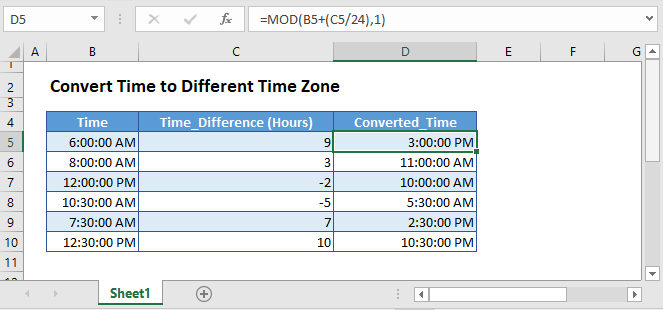To convert time values provided in one time zones to another time zone, you can use the following two methods.

## Simple Method

This is a simple way of converting time to a different time zone. Here, simply take the number of hours difference between the time zones, in column C, divide it by 24, and then add to the original time (column B):

``=B3+(C3/24)``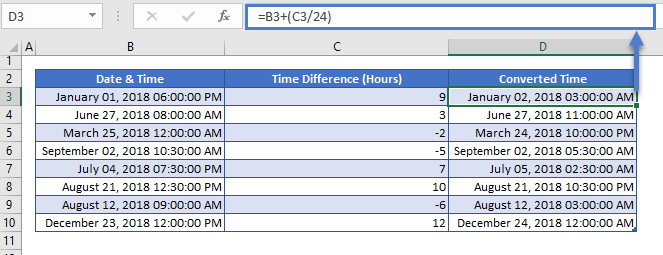This simple formula works well because, in Column B, the Date is provided along with the time. However, if only the time was provided, you could run into issues where the formula doesn’t work.

Excel can not handle negative times. So here we attempting to subtract for hours from 01:00, which results in an error: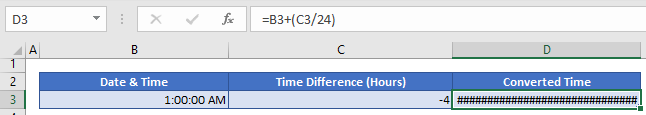## Foolproof Method

If only the time is given and we need to convert it to different time zone, then we’ll use MOD function like this:

``=MOD(B5+(C5/24),1)``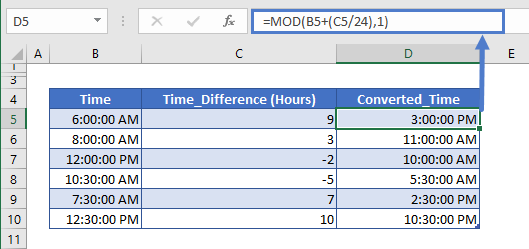The MOD function will return the remainder after a value is divided by its divisor. Also as Excel doesn’t show negative time values. So, if this formula (time + (hours/24)) returns a negative decimal value, then MOD will return the reciprocal value. E.g. if the formula returns -0.375 value, the MOD will return 0.625 i.e. equivalent to 3 PM.

In Excel dates and times are stored as the date-time serial number, where the integer part represents the date and the decimal part represents the time. E.g. in Excel, the May 22nd, 2017 3:00 AM has a stored value of 42877.125, where 42877 represents the date and 0.125 represents the time.

And in both formulas (Hours/24) is used to convert the adjusted hours into fractions before adding to the time value. Because as stated above, time is stored in decimal value in Excel. And there are 24 hours in a day, so 1 hour is 1/24 of the day or 0.41667.

## Time Zone Conversion in Google Sheets

The time zones conversion methods work exactly the same in Google Sheets as in Excel: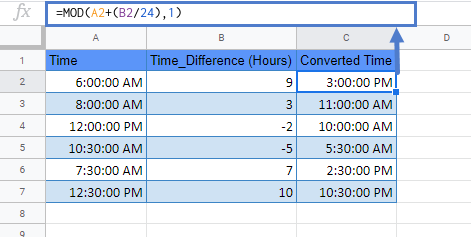### Excel Practice Worksheet

Practice Excel functions and formulas with our 100% free practice worksheets!